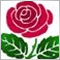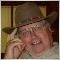Weighted Moving Average Different in MQL4 and MQL5?1083

For some reason the iMAOnArray() function for a linear-weighted moving average in MQL4 produces different results to the WMA calculation function found in examples for MQL5:

void CalculateLWMA(int rates_total,int prev_calculated,int begin,const double &price[])
{
int        i,limit;
static int weightsum;
double     sum;
//--- first calculation or number of bars was changed
if(prev_calculated==0)
{
weightsum=0;
limit=MAPeriod+begin;
//--- set empty value for first limit bars
for(i=0;i<limit;i++) Coppock[i]=0.0;
//--- calculate first visible value
double firstValue=0;
for(i=begin;i<limit;i++)
{
int k=i-begin+1;
weightsum+=k;
firstValue+=k*price[i];
}
firstValue/=(double)weightsum;
Coppock[limit-1]=firstValue;
}
else limit=prev_calculated-1;
//--- main loop
for(i=limit;i<rates_total;i++)
{
sum=0;
for(int j=0;j<MAPeriod;j++) sum+=(MAPeriod-j)*price[i-j];
Coppock[i]=sum/weightsum;
}
//---
}

Is it something wrong with this custom function or with iMAonArray()? Is there any other way to substitute MQL4 iMAonArray() than with such custom functions?

Documentation on MQL5: Standard Constants, Enumerations and Structures / Chart Constants / Types of Chart Events
• www.mql5.com
Standard Constants, Enumerations and Structures / Chart Constants / Types of Chart Events - Documentation on MQL5588

enivid:

For some reason the iMAOnArray() function for a linear-weighted moving average in MQL4 produces different results to the WMA calculation function found in examples for MQL5:

Is it something wrong with this custom function or with iMAonArray()? Is there any other way to substitute MQL4 iMAonArray() than with such custom functions?

Check out this Migrate MT-4 to MT-5 article. The MT-5 IMAOnArray() version is lengthy. Hope it works OK.

Migrating from MQL4 to MQL5
• 2010.05.17
• Sergey Pavlov
• www.mql5.com
This article is a quick guide to MQL4 language functions, it will help you to migrate your programs from MQL4 to MQL5. For each MQL4 function (except trading functions) the description and MQL5 implementation are presented, it allows you to reduce the conversion time significantly. For convenience, the MQL4 functions are divided into groups, similar to MQL4 Reference.1083

The problem has been resolved and the topic can now be closed :).